# Logical Equivalence – Identity Law

Earlier you learned about the logical equivalence and how two or more compound prepositions makes a tautology and prove their equivalence.

There are many well-known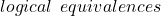, so first one is identity law. We call it law because the same logic is applied in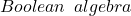which is another branch of mathematics, that studies and understand logic in terms of algebra. But that is out of scope for this article.

## Duality

As I mentioned earlier in a previous article, that duality applies to logical equivalences. A logical equivalence is made of compound statements which has a dual. So, every equivalence has a dual obtain by changing the connectives and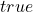to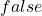orto true if any.

\begin{aligned}
&P \wedge Q \vee T \\\\
&Dual \hspace{5px} is \\\\
&P \vee Q \wedge F
\end{aligned}

## Understanding The Identity Law

Suppose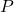is a statement “I ate breakfast” with some other true statement which we don’t know. The truth value of other statement is always, so we don’t need a variable for that, instead we call it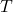which stands for.

\begin{aligned}
&P \wedge T \equiv P\\
&P \vee F \equiv P
\end{aligned}

In the first equivalence of identity law, whenis, then bothand the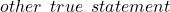giveswhich is same asbecuase truth value ofis.

Ifis, the compound statement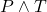becomeswhich is same as. The equivalence is valid and a tautology.

The second equivalence states that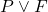is equivalent to. The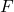stands formeaning we are referring to some statement which is always going to be false, and which is why we don’t use a variable.

Ifvalue is, output of compound statement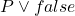isas per the working of disjunction, and equivalence holds.

Ifis, then compound statementorisand equivalent to.
The equivalence is true again.

## Translating Identity Law To English

If we translate the identity law into English.

P and True equivalent to P becomes
"I ate breakfast and Human being need food to survive"
is equivalent to saying "I ate breakfast".
P and True equivalent to P becomes
"I ate breakfast and Human being need food to survive"
is equivalent to saying "I ate breakfast".

The second statment does not need to be mentioned explicitly. It is always going to be true. Therefore, the equivalence is true regardless of second statement.

If I did not eat breakfast, the second statement does not make a difference. The equivalence is.

 (P or False) is equivalent to P can translate to "I at breakfast or I can survive without food and water" which is same as "I ate breakfast"

Similarly, and the false statement “I can survive without food and water” is irrelevant.

The second statement does not matter here because if I ate breakfast the compound statementis. It is same as saying “I ate breakfast”. The equivalence holds. Suppose “I did not eat breakfast”, even then the equivalence holds, and it is a tautology.

## Truth Table For Identity Law

It would be easier to visualize Identity law with truth table. So first we make a truth table with 2 rows because the compound preposition has only one variable which isand other entity is either true or false.

The columnandentries match with columnwhich means the logical equivalence of identity law is valid and hence proved.